### Moving and copying formulas and referenced cells

Formula references change in a logical manner when you move cells that contain formulas or cells referenced by formulas. For additional information, see “Rules for moving formulas and referenced cells.”

You can move formulas to any spreadsheet in a notebook. When you move a formula, the formula readjusts to calculate the cells relative to its new location. If you want the formula to calculate the cells it referenced before the move, you must set the formula to calculate absolute cell addresses. For additional information, see “Setting cell addresses.”

You can copy the formulas you create in Quattro Pro. When you copy data by dragging and dropping the cells, the formulas adjust to correspond with their new positions. When you copy formulas with copy and paste, the formulas adjust to the new location if it includes values to use in the formula.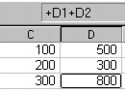However, if you copy the formula to D3, and D1 and D2 are empty, you are alerted that the formula is using an empty cell and provided with an option to fix it. Quattro Pro will find the original data associated with the copied formula and make the reference absolute.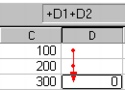A red-lined arrow points to the location where Quattro Pro “guesses” you want to get the data to use in the formula. If this location includes empty cells, Quattro Pro reports an error.

You can also have Quattro Pro check your cell references while you work.

#### To move a formula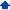1 Select a formula.
 2 Click Edit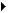Cut.
 3 Select the cell in which to paste the formula.
 4 Click EditPaste.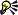• You can also move a formula by selecting the formula cell, moving the cursor to the edge of the cell until the pointer changes to a four-way arrow, and dragging the formula to a new location.

#### To copy a formula1 Select a formula.
 2 Click EditCopy.
 3 Click the cell in which to paste the formula.
 4 Click EditPaste.
 5 In the Cell reference checker dialog box (if appearing), click one of the following buttons:
 • Close — keeps the adjusted formula
 • Fix it — uses the original formula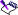• By default, when you copy formulas containing cell names, the cell names in the new location use absolute cell referencing. To force Quattro Pro to use relative cell referencing, precede the cell name with a tilde (~). For example, AGE would be an absolute cell reference, while ~AGE would be a relative cell reference. For more information about c ell names, see “Naming cells.”• You can also copy a formula by selecting the formula cell, moving the cursor to the edge of the cell until the pointer changes to a four-way arrow, and holding down Ctrl while dragging the formula to a new location.

#### To check cell references1 Click ToolsSettings.
 2 Click General.
 3 Enable the Cell reference checker check box.